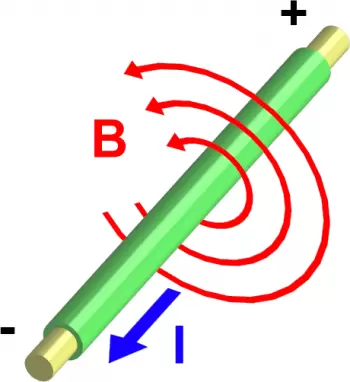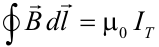# State Ampere’s Circuital Law: Formula, Uses, and ExamplesAmpère's law is one of the fundamental laws of classical electrodynamics. This law was formulated by the physicist and mathematician André-Marie Ampère (1775-1836) in 1826.

It was one of the main theorems of electromagnetism that is usually considered to be the magnetic counterpart of Gauss's theorem.

This law allows us to determine the magnetic field using electric currents.

The electric field is conservative, which implies that its circulation along a closed line is zero.

The magnetic field lines generated by a rectilinear current are circular. Unlike electric or gravitational field lines, they have no beginning or end. However, magnetic fields are not conservative; therefore, the circulation along a closed line is not zero and is given by Ampère's law.

## What States Ampere's Circuital Law?

This law states that “the line integral of the magnetic field created surrounding closed-path equals the number of times the algebraic sum of currents passing through the loop.”

In other words, Ampère's law determines that the circulation of the magnetic field along a closed path is equal to the algebraic sum of the currents crossing the surface delimited by the closed loop. In addition, this value must be multiplied by the medium's permittivity, Specifically for the vacuum.

## What Is Ampere's Circuital Law Formula?

The following equation can represent Ampère's law:In this formula for calculating the magnetic field, the integral represents the circulation of the field lines along a closed path and:

• μ0 is the permeability of the vacuum

• dl is a vector tangent to the path chosen at each point

• IT is the net current intensity that passes through the surface delimited by the path. It will be positive or negative depending on the direction in which it crosses the surface.

## Applications for Ampère's Law

Ampère's law allows us to know the magnetic field of:

• A solenoid

This law can be used for electromagnets, generators, transformers, and motors.

## Applications for a Solenoid

The law discovered by André Marie Ampère can be seen applied to a solenoid in order to find the magnetic field found inside it. This magnetic field is proportional to the applied current and the number of turns times its length. The electromagnet is an application where we can clearly see this law since the magnetic field is equal to the sum of the magnetic fields of the said spiral, and this can be calculated with Ampère's law.

In addition to applying it to solenoids, they can also be applied to toroids.

## Example of Application of Ampere's Law: the Electromagnet

An electromagnet is a type of magnet that is activated when an electrical current flows through it. Usually, electromagnets are made up of many turns of wire very close to each other.

If the ends of this wire are connected to a potential difference, electric current flows through it, and a magnetic field is generated.

This magnetic field is equivalent to the sum of the magnetic fields of each loop and can be calculated by applying Ampère's law.

## Attraction Force Between Conductors with Electric Current

Two current-carrying wires influence each other. Parallel and rectified electric currents attract each other, and parallel and opposite currents repel.

It can be understood in terms of the Lorentz force: the current in one wire generates a magnetic field which, due to Lorentz's law, produces a force on the moving charges in the other wire, and therefore both wires feel a mutual influence.

## What Are Maxwell's Laws?

After discovering Ampère's law, James Clerk Maxwell summarized the entire theory of electromagnetism in four equations: Maxwell's laws.

Ampere's law, with an extension for a time-dependent dielectric shift, is one of them. However, Maxwell's laws are often written in differential form, not with integrals as before, but with equations that give relationships between the derivatives of the electric and magnetic fields.

Author:

Published: September 15, 2021
Last review: September 15, 2021